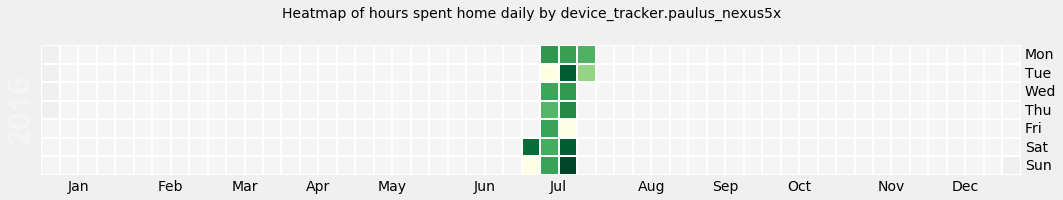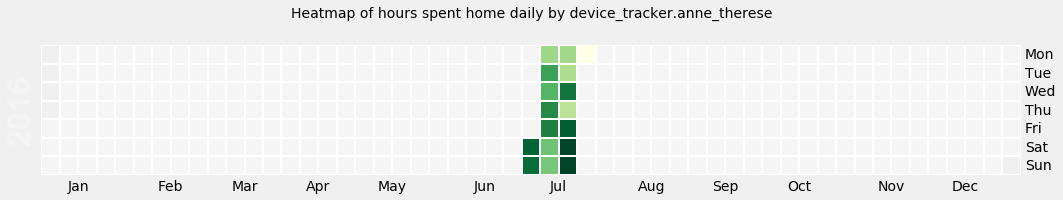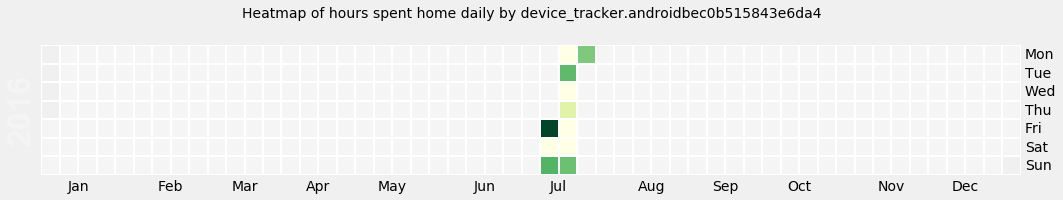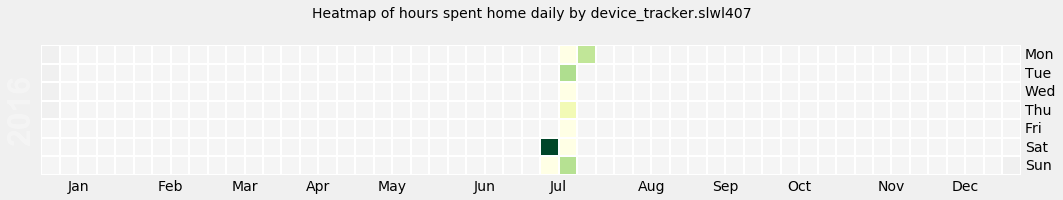In :
%matplotlib inline
from sqlalchemy import create_engine, text
from datetime import timedelta
import pandas as pd
import numpy as np
import matplotlib.pyplot as pltt
import calmap

# setting up a visual style for PyPlot, much better than the standard
pltt.style.use('fivethirtyeight')

# Your database url as specified in configuration.yaml
# If using default settings, it's \
# sqlite:///<path to config dir>/home-assistant_v2.db
DB_URL = "sqlite:///./home-assistant_v3.db"
engine = create_engine(DB_URL)

# let's get a list of distinct entity_ids from domain 'device tracker'
entitystmt = text("select distinct entity_id from states where \
domain='device_tracker'")
entitiesquery = engine.execute(entitystmt)
entitylist = list(entitiesquery.fetchall())

# now let's loop through the entitylist to generate plots for each entity
for entity in entitylist:

# sql query pulling each state for a given entity, joining in on itself \
# in order to get the time of next state change
stmt = text("select distinct case when t1.state='home' then 1 else 0 end as \
HomeBit, t1.last_changed, (select  last_changed from states t2 where \
t2.state_id> t1.state_id and entity_id=:entity_filter order by \
t2.state_id limit 1) as next_change from states t1 where \
entity_id=:entity_filter")

# bind entity parameter to query
stmt = stmt.bindparams(entity_filter=entity)

# execute the SQL statement
devicestatesquery = engine.execute(stmt)

# get rows from query into a pandas dataframe
devicestatesDF = pd.DataFrame(devicestatesquery.fetchall())

# name the dataframe rows for usability
devicestatesDF.columns = ['HomeBit',  'last_changed', 'next_change']

# create a dataframe with an index ranging from the min to the max\
# last_changed timestamp from our query
dates = pd.DataFrame(index=pd.date_range(min(pd.to_datetime(
devicestatesDF['last_changed']).dt.date.unique()),
max(pd.to_datetime(devicestatesDF['last_changed']).dt.date.unique()
)-timedelta(days=1)))

# create a HomeTime column with a zero timedelta value
dates['HomeTime'] = timedelta(days=0)

# create a last_changed_date column with only the date portion of \
# last_changed value
devicestatesDF['last_changed_date'] = pd.to_datetime(devicestatesDF
['last_changed']).apply(lambda x: x.date())

# grab the index values of our dates dataframe
dateslist_DATE = pd.to_datetime(dates.index.values.tolist())

# start processing for each date
for date in dateslist_DATE:

# create a filtered dataframe where the last_changed_date column \
# matches the date currently being processed
datedf = devicestatesDF.loc[devicestatesDF['last_changed_date'] ==
date.date()]

# the filtered dataframe will retain the indexes, so let's reset them
datedf.reset_index(inplace=True)

# initialize our "counter" as a 0 timedelta
datetimehomesum = pd.Timedelta(hours=0, unit='us')

# start processing the filtered dataframe row by row
for item in datedf.index:

# if this is the first row of current day
if item == 0:

# and the first row of this day indicates that the device \
# wasn't home, it must've been home for whatever time since \
# the beginning of current day
if datedf.ix[item]['HomeBit'] == 0:
datetimehomesum += (pd.to_datetime(datedf.ix[item]
['last_changed'])-pd.to_datetime(pd.to_datetime
(datedf.ix[item]['last_changed']).date()))*1

# if there's more rows to be processed in this day, \
# calculate the home value from first timestamp to second
if len(datedf.index) > 1:
datetimehomesum += (pd.to_datetime(datedf.ix[item]
['next_change'])-pd.to_datetime(datedf.ix[item]
['last_changed']))*datedf.ix[item]['HomeBit']

# if the first row of this day indicates that the device was\
# home, calculatee the home value for the first timestamp as \
# usual
else:
datetimehomesum += (pd.to_datetime(datedf.ix[item]
['next_change'])-pd.to_datetime(datedf.ix[item]
['last_changed']))*datedf.ix[item]['HomeBit']

# if this is the last row of this day, calculate the home value\
# until beginning of next day
elif item == len(datedf.index)-1:
datetimehomesum += (pd.to_datetime(((pd.to_datetime(datedf.ix
[item]['last_changed']))+(timedelta(days=1))).date()) -
pd.to_datetime(datedf.ix[item]['last_changed']))\
* datedf.ix[item]['HomeBit']

# otherwise calculate the home value as usual
else:
datetimehomesum += (pd.to_datetime(datedf.ix[item]
['next_change'])-pd.to_datetime(datedf.ix[item]
['last_changed']))*datedf.ix[item]['HomeBit']

# set the value of our dates dataframe at index of current date\
# to the calculated home value for this day
dates.loc[date] = datetimehomesum

# convert index of our dates dataframe to datetime
dates.index = pd.to_datetime(dates.index, box=True)

# convert each value in our dates dataframe from an hour timedelta \
# to a float
dates = dates.apply(lambda x: x/np.timedelta64(1, 'h'))

# create a series from our dates dataframe (necessary for calmap)
datesseries = dates['HomeTime']

# create a calendar map
entityplot = calmap.calendarplot(datesseries, cmap='YlGn', fig_kws=dict(figsize=(15, 3)),
linecolor='white')

# set the plot title to match our current entity name
entityplot.suptitle('Heatmap of hours spent home daily by {}'.format(entity))
pltt.show()

C:\Users\anton.kireyeu\Downloads\WinPython-64bit-3.4.4.2\python-3.4.4.amd64\lib\site-packages\calmap\__init__.py:294: FutureWarning: how in .resample() is deprecated
the new syntax is .resample(...).sum()
by_day = data.resample('D', how=how)